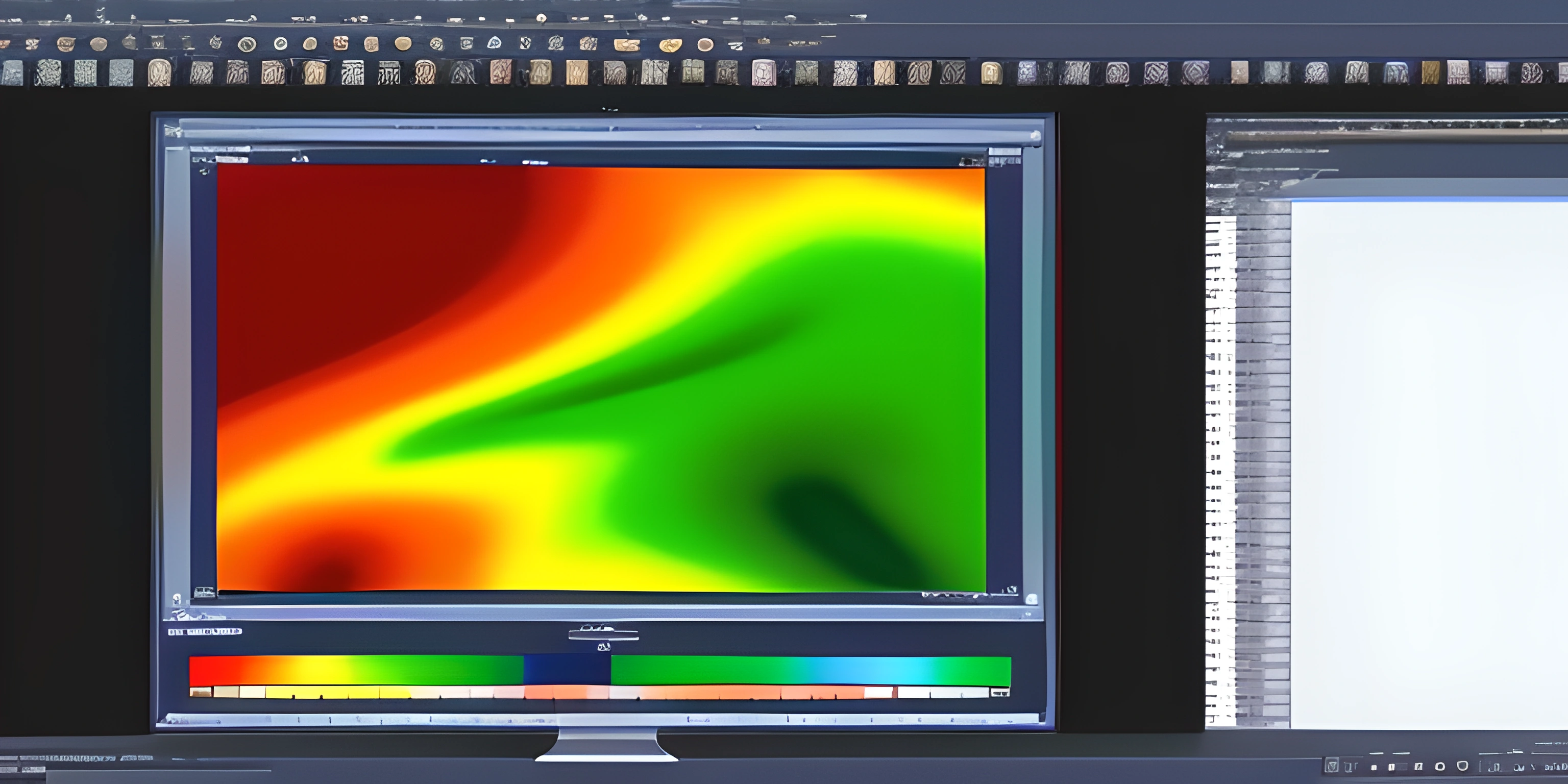# Matlab ColormapsNote: this page has been created with the use of AI. Please take caution, and note that the content of this page does not necessarily reflect the opinion of Cratecode.

MATLAB is known for its strong visualization capabilities, and one of the ways to enhance your plots and visualizations is through the use of colormaps. Colormaps are used to map data values to colors, which can be especially useful when visualizing data on a 2D or 3D plot. In this article, we'll explore the different colormaps available in MATLAB and how to use them effectively.

## Default Colormap: Parula

MATLAB has a default colormap called `parula`, which is a perceptually uniform colormap. This means that the color transitions are designed to be smooth and uniform, making it easier to identify trends and patterns in the data. Here's a simple example of using the default `parula` colormap:

``````% Create a matrix
M = magic(7);

% Create a 2D plot using the default colormap
imagesc(M);
colorbar;``````

To change the colormap, you can use the `colormap` function and pass in the name of the colormap you wish to use.

## Available Colormaps

MATLAB has a variety of built-in colormaps to choose from. Here's a list of some popular options:

1. `jet`: A popular colormap used in many scientific visualizations, but it's not recommended for accurate data representation due to its non-uniform color transitions.
2. `hsv`: A colormap that transitions through the colors of the rainbow, based on the hue-saturation-value (HSV) color model.
3. `hot`: A colormap that transitions from black to red to yellow to white, giving the appearance of heat.
4. `cool`: A colormap that transitions from cyan to magenta, creating a cool color palette.
5. `gray`: A grayscale colormap, ranging from black to white.
6. `bone`: A colormap with a higher color density around the middle values, simulating an X-ray image.
7. `copper`: A colormap that transitions from dark to light copper tones.

You can apply any of these colormaps to your plot by passing their name as an argument to the `colormap` function:

``````% Create a matrix
M = magic(7);

% Create a 2D plot using the 'jet' colormap
imagesc(M);
colormap('jet');
colorbar;``````

## Creating Custom Colormaps

If the built-in colormaps don't suit your needs, you can create a custom colormap. A colormap is an n-by-3 matrix, where n is the number of colors and each row represents an RGB color value between 0 and 1:

``````% Create a custom colormap
custom_colormap = [0 0 0;       % Black
1 0 0;       % Red
0 1 0;       % Green
0 0 1;       % Blue
1 1 1];      % White

% Create a 2D plot using the custom colormap
imagesc(M);
colormap(custom_colormap);
colorbar;``````

You can also combine built-in colormaps to create a custom one:

``````% Combine 'hot' and 'cool' colormaps
combined_colormap = [colormap('hot'); colormap('cool')];

% Create a 2D plot using the combined colormap
imagesc(M);
colormap(combined_colormap);
colorbar;``````

## FAQ

### What is a colormap?

A colormap is a mapping of data values to colors, used to enhance the visualization of data on plots. Colormaps are essential tools in MATLAB to help create easily interpretable 2D and 3D plots.

### What is the default colormap in MATLAB?

The default colormap in MATLAB is called `parula`. It is a perceptually uniform colormap designed to display smooth and uniform color transitions.

### How can I change the colormap in MATLAB?

To change the colormap in MATLAB, use the `colormap` function and pass in the name of the colormap you want to use, like `colormap('jet')`.

### Can I create custom colormaps in MATLAB?

Yes, you can create custom colormaps in MATLAB by defining an n-by-3 matrix, where n is the number of colors and each row represents an RGB color value between 0 and 1. You can then pass this matrix to the `colormap` function.